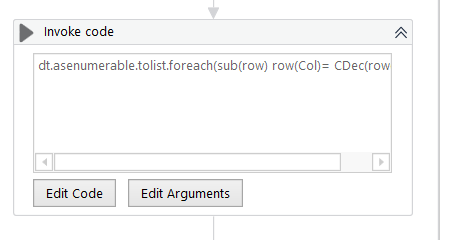# Skip Empty Row in DataTable

Hello,

I Have this scenario. I have DT, and I want to convert the value on the specific column from string to Decimal. it’s working fine if all the row is not empty, but it went to error when some row is empty.
I’m using invoke code to convert the row
dt.asenumerable.tolist.foreach(sub(row) row(Col)= CDec(row(Col)))Thanks,

before invoke code could you add a condition if isnullorempty?

Can we have if statement inside the code, the thing is I’m not using for each row before invoke code

yes you can assgia the row value to a variable can check it is null, then choose to by pass it

``````dt.asenumerable.Where(function(row) not String.IsNullOrEmpty(row(Col).ToString)).tolist.foreach(sub(row) row(Col)= CDec(row(Col)))
``````

Thanks

It’s working perfectly, thanks @prasath_S